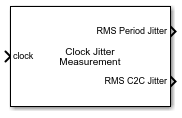# Clock Jitter Measurement

Measure jitter in periodic signals

• Library:
• Mixed-Signal Blockset / Utilities

•## Description

Use the Clock Jitter Measurement block to measure the RMS (root mean squared) periodic jitter in clock signals. You can also measure cycle-to-cycle (C2C) jitter and duty cycle distortion (DCD).

## Ports

### Input

expand all

Input clock signal, specified as a scalar. The input signal must have only one threshold crossing per period.

Data Types: `double`

### Output

expand all

Running RMS value of the period jitter calculated up to the current time step, returned as a scalar. Period jitter is the deviation in the cycle time of a clock signal with respect to the ideal period.

Data Types: `double`

Running RMS value of the cycle-to-cycle jitter calculated up to the current time step, returned as a scalar. Cycle-to-cycle jitter is the difference in the period between the two consecutive cycles of the clock.

#### Dependencies

To enable this port, select Cycle-2-Cycle (C2C) Jitter.

Data Types: `double`

Difference between the real duty cycle in percentage and the ideal 50% value, returned as a scalar.

#### Dependencies

To enable this port, select Duty Cycle Distortion (DCD).

Data Types: `double`

## Parameters

expand all

Frequency of the input clock signal, specified as a positive real scalar in Hz. Clock Frequency is used to calculate the ideal value of the period of the input signal.

#### Programmatic Use

 Block parameter: `Frequency` Type: character vector Values: positive real scalar Default: `1e6`

Threshold signal level to calculate the rising and falling edge of the signal, specified as a real scalar.

#### Programmatic Use

 Block parameter: `Threshold` Type: character vector Values: real scalar Default: `0`

Select to calculate the RMS period jitter. This option is selected by default.

Period jitter is the deviation in the cycle time of a clock signal with respect to the ideal period.

Select to calculate the RMS cycle-to-cycle (C2C) jitter. This option is deselected by default.

Cycle-to-cycle jitter is the difference in the period between the two consecutive cycles of the clock.

Select to calculate the duty cycle distortion for each cycle. This option is deselected by default.

Duty cycle distortion is the difference between the real duty cycle in percentage and the ideal 50% value.

Select the simulation mode. This choice affects the simulation performance.

Simulating the model using the `Code generation` method requires additional startup time, but the subsequent simulations run faster. Simulating the model using the `Interpreted execution` method may reduce the startup time, but the subsequent simulations run slower. For more information, see Simulation Modes.

## Version History

Introduced in R2021a